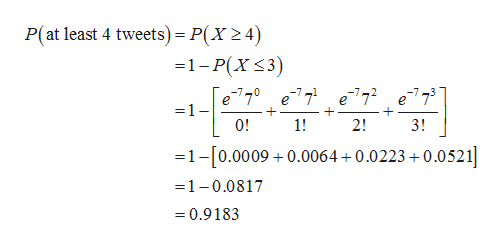Over 500 million tweets are sent per day (Digital Marketing Ramblings website, December 15, 2014). Assume that the number of tweets per hour follows a Poisson distribution and that Bob receives on average 7 tweets during his lunch hour.a.  What is the probability that Bob receives no tweets during his lunch hour (to 4 decimals)? b.  What is the probability that Bob receives at least 4 tweets during his lunch hour (to 4 decimals)? For this question, if calculating the probability manually make sure to carry at least 4 decimal digits in your calculations. c.  What is the expected number of tweets Bob receives during the first 30 minutes of his lunch hour (to 1 decimal)? d.  What is the probability that Bob receives no tweets during the first 30 minutes of his lunch hour (to 4 decimals)?

Question

Over 500 million tweets are sent per day (Digital Marketing Ramblings website, December 15, 2014). Assume that the number of tweets per hour follows a Poisson distribution and that Bob receives on average 7 tweets during his lunch hour.

a.  What is the probability that Bob receives no tweets during his lunch hour (to 4 decimals)?

b.  What is the probability that Bob receives at least 4 tweets during his lunch hour (to 4 decimals)? For this question, if calculating the probability manually make sure to carry at least 4 decimal digits in your calculations.

c.  What is the expected number of tweets Bob receives during the first 30 minutes of his lunch hour (to 1 decimal)?

d.  What is the probability that Bob receives no tweets during the first 30 minutes of his lunch hour (to 4 decimals)?

Step 1

Hey, since multiple sub parts are posted, we will answer first three subparts according to our policy. If you want any specific subpart to be answered, then please submit that part only or specify the subpart in your message.

Step 2

a.

Here, number of tweets per hour follows a Poisson distribution. That is, X ~ Poisson (λ = 7).

The probability that Bob receives no tweets during his lunch hour is calculated as follows:

Step 3

b.

Here, X ~ Poisson (λ = 7).

The probability that Bob receives at least 4 tweets during ...help_outlineImage TranscriptioncloseP(at least 4 tweets) = P(X2 4) -1-P(X 3) e77e77e77e773 =1 + + 0! 1! 2! 3! 1-0.0009 0.0064+0.0223 +0.0521 =1-0.0817 0.9183 fullscreen

Want to see the full answer?

See Solution

Want to see this answer and more?

Our solutions are written by experts, many with advanced degrees, and available 24/7

See Solution
Tagged in

Other